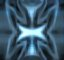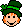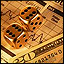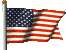The time is now 1:57 am

You last visited
August 19, 2022, 12:01 am

All times shown are
Eastern Time (GMT-5:00)# Using playing cards to pick numbersPrev TopicNext Topic

Page 1 of 1
•Zeta Reticuli Star System
United States
Member #30,469
January 17, 2006
11,679 Posts
Offline

Found this on a search for "playing cards + lotto numbers".

This fits like a glove for a 6/52 game, but use it anyway you wish:

 SPADES HEARTS CLUBS DIAMONDS A=1 A=14 A=27 A=40 2=2 2=15 2=28 2=41 3=3 3=16 3=29 3=42 4=4 4=17 4=30 4=43 5=5 5=18 5=31 5=44 6=6 6=19 6=32 6=45 7=7 7=20 7=33 7=46 8=8 8=21 8=34 8=47 9=9 9=22 9=35 9=48 10=10 10=23 10=36 10=49 J=11 J=24 J=37 J=50 Q=12 Q=25 Q=38 Q=51 K=13 K=26 K=39 K=52

Those who run the lotteries love it when players look for consistency in something that's designed not to have any. So many systems, so many theories, so few jackpot winners.There is one and only one 'proven' system, and that is to book the action. No matter the game, let the players pick their own losers.

•50
Chief Bottle Washer
New Jersey
United States
Member #1
May 31, 2000
26,964 Posts
Online

<Moved to Lottery Systems forum>

Please post in the appropriate forum ... thank you.

•Zeta Reticuli Star System
United States
Member #30,469
January 17, 2006
11,679 Posts
Offline

Sorry, Todd...I wasn't sure where to put this one.

Maybe you get get a playing card company to print some up with a LP logo on the cardbacks and sell them in the LP Gift Shop!

Those who run the lotteries love it when players look for consistency in something that's designed not to have any. So many systems, so many theories, so few jackpot winners.There is one and only one 'proven' system, and that is to book the action. No matter the game, let the players pick their own losers.

•Oklahoma
United States
Member #45,969
September 1, 2006
5,000 Posts
Offline

Quote: Originally posted by Coin Toss on Dec 19, 2008

Sorry, Todd...I wasn't sure where to put this one.

Maybe you get get a playing card company to print some up with a LP logo on the cardbacks and sell them in the LP Gift Shop!

Hey Todd, this is not a bad idea, whther this works or not. What are the chances?GOOD LUCK EVERYONE

•long, island
Bahamas
Member #62,179
June 20, 2008
3,681 Posts
Offline

Quote: Originally posted by Coin Toss on Dec 18, 2008

Found this on a search for "playing cards + lotto numbers".

This fits like a glove for a 6/52 game, but use it anyway you wish:

 SPADES HEARTS CLUBS DIAMONDS A=1 A=14 A=27 A=40 2=2 2=15 2=28 2=41 3=3 3=16 3=29 3=42 4=4 4=17 4=30 4=43 5=5 5=18 5=31 5=44 6=6 6=19 6=32 6=45 7=7 7=20 7=33 7=46 8=8 8=21 8=34 8=47 9=9 9=22 9=35 9=48 10=10 10=23 10=36 10=49 J=11 J=24 J=37 J=50 Q=12 Q=25 Q=38 Q=51 K=13 K=26 K=39 K=52

some months back i did try this twice one number never came and the other took about a month but it came str8 thinking about trying it again.

•50
Chief Bottle Washer
New Jersey
United States
Member #1
May 31, 2000
26,964 Posts
Online

Quote: Originally posted by Coin Toss on Dec 19, 2008

Sorry, Todd...I wasn't sure where to put this one.

Maybe you get get a playing card company to print some up with a LP logo on the cardbacks and sell them in the LP Gift Shop!

I agree, that sounds pretty neat.

Check the State Lottery Report Card

Sign the Petition for True Lottery Drawings
Help eliminate computerized drawings!

•25
North Carolina
United States
Member #59,853
March 29, 2008
52,468 Posts
Offline

Quote: Originally posted by Todd on Dec 19, 2008

I agree, that sounds pretty neat.

I like that idea too!

https://youtu.be/UvM2Cmi-YR

George Micheal is singing in heaven now & the angels are cheering him on!

https://youtu.be/fDxzQJaA228

George & Aretha are still singing in heaven in the link above!

Page 1 of 1
Subscribe to this topic
Guest